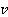### Create an Account

Home / Questions / Two masses m1 and m2 are connected by a massless spring of spring constant k The spring is...

# Two masses m1 and m2 are connected by a massless spring of spring constant k The spring is at its equilibrium length and the masses are both at rest there is no gravitational field Suddenly m2 is

Two masses m1 and m2 are connected by a massless spring of spring constant k. The spring is at its equilibrium length and the masses are both at rest; there is no gravitational field. Suddenly m2 is given a velocity. Assume thatis so small that the two masses never collide in their subsequent motion. Describe the motion of both masses. What are their maximum and minimum separations?

Jun 16 2020 View more View LessSubscribe To Get Solution